TRIGONOMETRIC RATIOS OF ACUTE ANGLES

Example 1:

Find the value of sin 34o with the calculator rounded to four decimal places.  The calculator must be in degree mode!

Input: sin(34) ENTER

NOTE:  Left parenthesis will open when we activate the trigonometric function buttons on the calculator.  After we type in the angle also called the argument, we MUST type in the right parenthesis, namely ")", before we press ENTER.

sin 34o0.5592

Please note that the value of sin 34o is an irrational number. That is, it has an infinite number of decimal places!

Example 2:

Find the value of cos 75o with the calculator rounded to four decimal places. The calculator must be in degree mode!

Input: cos(75) ENTER

cos 75o0.2588

Please note that the value of cos 75o is an irrational number. That is, it has an infinite number of decimal places!

Example 3:

Find the value of tan 84o with the calculator rounded to four decimal places.  The calculator must be in degree mode!

Input: tan(84) ENTER

NOTE:  Left parenthesis will open when we activate the trigonometric function buttons on the calculator.  After we type in the angle also called the argument, we MUST type in the right parenthesis, namely ")", before we press ENTER.

tan 84o9.5144

Please note that the value of tan 84o is an irrational number. That is, it has an infinite number of decimal places!

Example 4:

Find the value of csc 39o with the calculator rounded to three decimal places.

Calculators only have a sin, cos, and tan key.  Therefore, we MUST know and use the Reciprocal IdentityWe also must make sure that the calculator is in degree mode.

Input: 1sin(39) ENTER

csc 39o1.589

Please note that the value of csc 39o is an irrational number. That is, it has an infinite number of decimal places!

Example 5:

Find the value of sec 13o with the calculator rounded to three decimal places.
We MUST use the Reciprocal IdentityWe also must make sure that the calculator is in degree mode.

Input: 1cos(13) ENTER

sec 13o1.026

Please note that the value of sec 13o is an irrational number. That is, it has an infinite number of decimal places!

Example 6:

Find the value of cot 64o with the calculator rounded to three decimal places.

We can use the Reciprocal Identityor the Quotient IdentityNOTE: When working with the calculator, it is always best to use the Quotient Identity when evaluating cotangent. Sometimes, the calculator gives incorrect results when the Reciprocal Identity is used.

We must make sure that the calculator is in degree mode.

Input: cos(64)sin(64) ENTER

cot 64o0.488

Please note that the value of cot 64o is an irrational number. That is, it has an infinite number of decimal places!

Example 7:

Use the calculator to find the value of tan 1 rounded to 3 decimal places.

Since there is no degree symbol attached to the angle, the calculator must be in radian mode! Please note that radians are not always expressed in terms of.

Input: tan(1) ENTER

tan 11.557

Please note that the value of tan 1 is an irrational number. That is, it has an infinite number of decimal places!

Example 8:

Use the calculator to find the value ofrounded to three decimal places.

Since there is no degree symbol attached to the angle, the calculator must be in radian mode!

Input: cos(8) ENTER

NOTE: Always use thesymbol on the calculator and not 3.14.Please note that the value ofis an irrational number. That is, it has an infinite number of decimal places!

Example 9:

Use the calculator to find the value of sec 1.4 rounded to three decimal places.

Since there is no degree symbol attached to the angle, the calculator must be in radian mode!

We MUST use the Reciprocal IdentityInput: 1cos (1.4) ENTER

sec 1.45.883

Please note that the value of sec 1.4 is an irrational number. That is, it has an infinite number of decimal places!

Example 10:

Use the calculator to find the value of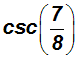rounded to three decimal places.

Since there is no degree symbol attached to the angle, the calculator must be in radian mode!

We MUST use the Reciprocal IdentityInput: 1(78 ) ENTERPlease note that the value ofis an irrational number. That is, it has an infinite number of decimal places!

Example 11:

Assume you forgot the EXACT value of sin 60o. Use a calculuator to find it.

The calculator must be in degree mode!

Input: sin(60) ENTER

sin 60o0.866 and we memorized that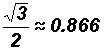given the sepcial angle 60o.

NOTE:

Some calculators have MATHPRINT. They display exact values of trigonometric ratios.

For example, if you have the TI-30XS Multiview Scientific Calculator, press the mode button and observe the modes CLASSIC or MATHPRINT in the last row of the display window. Use the arrow buttons (located under the display window) to highlight the MATHPRINT mode. Press enter and then clear.

Example 12:

Assume you forgot the EXACT value of sin 45o. Use a calculuator to find it.

The calculator must be in degree mode!

Input: sin (45) ENTER

sin 45o0.707 and we memorized that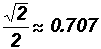given the special angle 45o.

Example 13:

Assume you forgot the EXACT value of tan 60o. Use a calculuator to find it.

The calculator must be in degree mode!

Input: tan (60) ENTER

tan 60o1.732 and we memorized that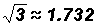given the special angle 60o.

Example 14:

Assume you forgot the EXACT value of tan 30o. Use a calculuator to find it.

The calculator must be in degree mode!

Input: tan (30) ENTER

tan 30o0.577 and we memorized that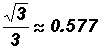given the special angle 30o.

Example 15:

Assume you forgot the EXACT value of. Use a calculuator to find it.

There is no degree symbol attached to the angle, therefore, the calculator must be in radian mode!

Input: cos(6) ENTER      Always use thesymbol on the calculator and not 3.14.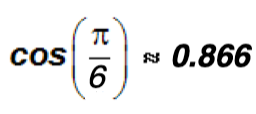and we memorized thatgiven the special angle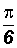(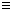30o).